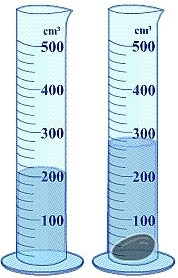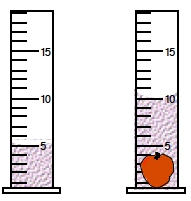## General Chemistry

Learn the toughest concepts covered in Chemistry with step-by-step video tutorials and practice problems by world-class tutors

1. Intro to General Chemistry

# Density of Non-Geometric Objects

The density of non-geometric objects can be determined using water displacement.

Calculating Density
1
concept

## Density of Non-Geometric Objects Concept and Example3m
Play a video:
Hey everyone. So when it comes to calculating the density of non geometric objects, we have to utilize what's called water displacement. Now, water displacement is just the amount of water moved out of the way, when an object is totally submerged. Now, here, we're gonna say what displacement can be used to determine the volume of non geometric objects. So we're going to look at this example here, it says, calculate the volume of the water displaced by the submerging of the object here. The volume of the given cylinders are in milliliters. So, if we take a look here, there's nothing inside of this first tube here. And we're gonna approximate what the volume is. It kind of looks like it touches around this line. So we're gonna say this is approximately 3.5 ml. Now, we've taken our non geometric object and placed it within the water. This causes a rise in the water level. We'll take a look here, we say that our new volume, it's a little bit harder to see. We're going to say that it's not quite six mls. We're gonna say here that It's approximately 5.8 m. So we're gonna say we have 5.8 MS after we've submerged the non geometric object. So the volume of the object would just be our Final volume of 5.8 ml - Our initial volume of 3. ml. So that would give me a volume of the non geometric object of 2.3 mL. So 2.3 mL would be my final answer for this example question
2
Problem

A piece of unknown solid weighs approximately 0.045 lbs. When a scientist places it in a glass beaker the water level increases from 200 mL to 260 mL. What is the density of the unknown solid in g/mL?3
Problem

If an irregularly shaped apple possesses a density of 0.96 g/cm3, what is its mass in milligrams? (The volume of the given cylinders are in mL).